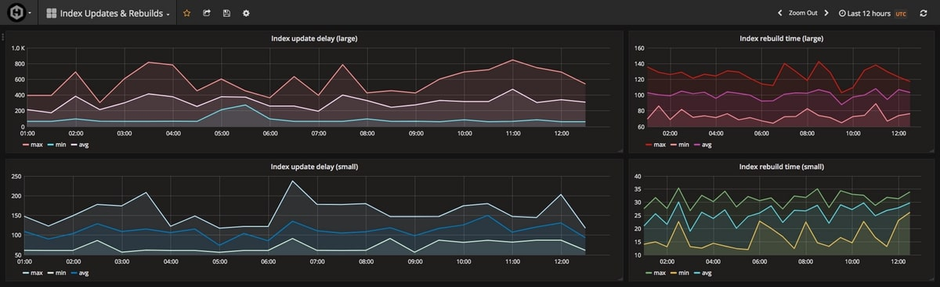# Data Views

MetricFire automatically produces different statistical views on the data you send, providing fast views on your metrics at the most appropriate resolution for viewing on your dashboard using Hosted Graphite.

## Views

The different views we keep by default for all our Graphite metrics are:

• :avg
Average (default)
• :sum
Sum of data points received during the timeframe of the current graph resolution. This changes depending on the zoom level.
Example: using:sum on a graph with data points drawn every 30 seconds will show the sum of all data points received in each 30-second period.
• :sumrate
The sum is divided by the number of seconds between data points, giving you a per-second rate.
:sum and :sumrate are the most common views to use with Counter metrics.
• :sum30 :sum60 :sumN
Provides the sum received over a given number of seconds, calculated from the :sumrate. E.g. ”:sum30” provides the sum over 30 seconds.
This is useful when you want a sum of a metric that is consistent regardless of what data resolution you are viewing.
When drawing a graph at a wider timescale there are more data points than available space to draw them. Using the ”:sumN” syntax lets you see an accurately scaled sum over a given number of seconds.
• :min
Minimum value
• :max
Maximum value
• :obvsrate
The observations are divided by the number of seconds in the collection period, giving you a per second rate.
• :90pct :95pct :99pct etc...
Want arbitrary percentile data? Just add the number after the colon followed by ‘pct’. It accepts values from 01 to 99. If you want 100th percentile you should be using ”:max”!

## Comparison to StatsD

The Hosted Graphite views aren’t perfectly analogous to statsd, but for general use, it accomplishes much the same thing.

• counting -> ‘:sum’ or ‘:obvs’
• rates -> ‘:sumrate’ or ‘:obvsrate’
• timers -> ‘:avg’ or ‘:min’ or ‘:max’

## Data Views and Graphite Functions

Graphite functions act on one or more data series, transforming them into a new data series. When using a graphite function it is important to note that:

• The data view specified in the query is not changed depending on the function. If you use the sumSeries() function, you will be summing the average values of each series unless you also use :sum in your query as well.
• The graph’s resolution can be changed by functions, but the resolution of the data series cannot. For example, summarize() allows you to group all the data points in a specified timeframe into one. Changing the zoom level of the graph won’t change the interval output by the summarize() function, but it may change the number of data points available to be